Courses

# One Dimensional Unsteady Conduction & Free & Forced Convection - MCQ Test 1

## 20 Questions MCQ Test RRB JE for Mechanical Engineering | One Dimensional Unsteady Conduction & Free & Forced Convection - MCQ Test 1

Description
This mock test of One Dimensional Unsteady Conduction & Free & Forced Convection - MCQ Test 1 for Mechanical Engineering helps you for every Mechanical Engineering entrance exam. This contains 20 Multiple Choice Questions for Mechanical Engineering One Dimensional Unsteady Conduction & Free & Forced Convection - MCQ Test 1 (mcq) to study with solutions a complete question bank. The solved questions answers in this One Dimensional Unsteady Conduction & Free & Forced Convection - MCQ Test 1 quiz give you a good mix of easy questions and tough questions. Mechanical Engineering students definitely take this One Dimensional Unsteady Conduction & Free & Forced Convection - MCQ Test 1 exercise for a better result in the exam. You can find other One Dimensional Unsteady Conduction & Free & Forced Convection - MCQ Test 1 extra questions, long questions & short questions for Mechanical Engineering on EduRev as well by searching above.
QUESTION: 1

Solution:

Ans. (b)

QUESTION: 2

### Assertion (A): In lumped heat capacity systems the temperaturegradient within the system is negligible Reason (R): In analysis of lumped capacity systems the thermalconductivity of the system material is considered very highirrespective of the size of the system

Solution:

Ans. (a)

If Biot number (Bi) =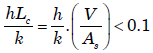then use lumped heat capacity
approach. It depends on size.

QUESTION: 3

### A thermocouple in a thermo-well measures the temperature of hot gas flowing through the pipe. For the most accurate measurement of temperature, the thermo-well should be made of:

Solution:

Ans. (a)

QUESTION: 4

A thermocouple in a thermo-well measures the temperature of hot gas flowing through the pipe. For the most accurate measurement of temperature, the thermo-well should be made of:

Solution:

Ans. (a)

QUESTION: 5

Assertion (A): During the temperature measurement of hot gas in aduct that has relatively cool walls, the temperature indicated by the thermometer will be lower than the true hot gas temperature.

Reason(R): The sensing tip of thermometer receives energy from the hot gas and loses heat to the duct walls.

Solution:

Ans. (a)

QUESTION: 6

A coolant fluid at 30°C flows over a heated flat plate maintained at aconstant temperature of 100°C. The boundary layer temperature distribution at a given location on the plate may be approximated asT = 30 + 70exp (–y) where y (in m) is the distance normal to the plate andT is in °C. If thermal conductivity of the fluid is 1.0 W/mK, the local convective heat transfer coefficient (in W/m2K) at that location will be:

Solution:

Ans. (b)
Given T = 30 + 70 e-y
or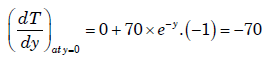We know that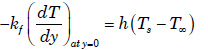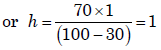QUESTION: 7

The ratio of energy transferred by convection to that by conduction iscalled

Solution:

Ans. (b)

QUESTION: 8

The ratio of energy transferred by convection to that by conduction iscalled

Solution:

Ans. (b)

QUESTION: 9

The ratio of energy transferred by convection to that by conduction iscalled  ]

Solution:

Ans. (b)

QUESTION: 10

Consider a hydrodynamically fully developed flow of cold air through a heated pipe of radius ro. The velocity and temperature distributions in the radial direction are given by u(r) and T(r) respectively. If um, is the mean velocity at any section of the pipe, then the bulk-mean temperature at that section is given by:

Solution:

Ans. (d) Bulk-mean temperature =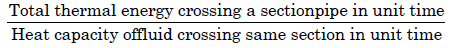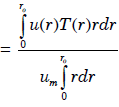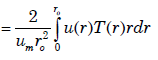QUESTION: 11

The velocity and temperature distribution in a pipe flow are given by u(r) and T(r). If um is the mean velocity at any section of the pipe, the bulk mean temperature at that section is:

Solution:

Ans. (d) Bulk temperature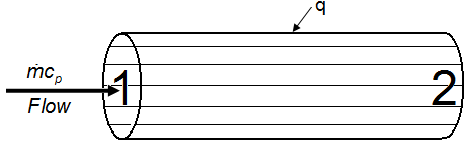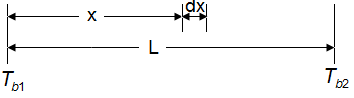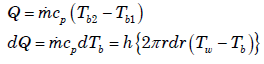• The bulk temperature represents energy average or ‘mixing cup’ conditions.
• The total energy ‘exchange’ in a tube flow can be expressed in terms of a bulk temperature difference.
QUESTION: 12

Assertion (A): When heat is transferred from a cylinder in cross flow to an air stream, the local heat transfer coefficient at the forwar dstagnation point is large.
Reason (R): Due to separation of the boundary layer eddiescontinuously sweep the surface close to the forward stagnation point.

Solution:

Ans. (b)

QUESTION: 13

Consider the following statements:
If a surface is pock-marked with a number of cavities, then ascompared to a smooth surface.
1. Radiation will increase
2. Nucleate boiling will increase
3. Conduction will increase
4. Convection will increaseOf these statements:

Solution:

Ans. (b) If coefficient of friction is increased radiation will decrease.

QUESTION: 14

Assertion (A): All analyses of heat transfer in turbulent flow musteventually rely on experimental data.
Reason (R): The eddy properties vary across the boundary layer and noadequate theory is available to predict their behaviour.

Solution:

Ans. (a)

QUESTION: 15

In the case of turbulent flow through a horizontal isothermal cylinderof diameter 'D', free convection heat transfer coefficient from thecylinder will:

Solution:

Ans. (a)

QUESTION: 16

For laminar flow over a flat plate, the local heat transfer coefficient 'hx'varies as x-1/2, where x is the distance from the leading edge (x = 0) ofthe plate. The ratio of the average coefficient 'ha' between the leading edge and some location 'A' at x = x on the plate to the local heat transfer coefficient 'hx' at A is

Solution:

Ans. (b) Here at

x = 0, h0 = h and at ,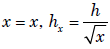Average coefficient =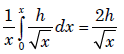Therefore ratio =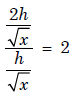QUESTION: 17

For fully-developed turbulent flow in a pipe with heating, the Nusselt number Nu, varies with Reynolds number Re and Prandtl number Pr as

Solution:

Ans. (c)

QUESTION: 18

Natural convection heat transfer coefficients over surface of a verticalpipe and vertical flat plate for same height and fluid are equal. Whatis/are the possible reasons for this?
1. Same height
2. Both vertical
3. Same fluid
4. Same fluid flow pattern

Select the correct answer using the code given below:

Solution:

Ans. (d) Same height, both vertical and same fluid everything

QUESTION: 19

Which one of the following statements is correct?
The non-dimensional parameter known as Stanton number (St) isused in

Solution:

Ans. (a)

QUESTION: 20

Which one of the following statements is correct?
The non-dimensional parameter known as Stanton number (St) isused in

Solution:

Ans. (a)